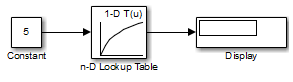## Create a Logarithm Lookup Table

Suppose you want to approximate the common logarithm (base 10) over the input range [1, 10] without performing an expensive computation. You can perform this approximation using a lookup table block as described in the following procedure. (If you want to skip the procedure, you can open the model directly.)

1. Copy the following blocks to a Simulink® model:

• One Constant block to input the signal, from the Sources library

• One n-D Lookup Table block to approximate the common logarithm, from the Lookup Tables library

• One Display block to display the output, from the Sinks library

2. Assign the table data and breakpoint data set to the n-D Lookup Table block:

1. In the Number of table dimensions field, enter `1`.

2. In the Table data field, enter ```[0 .301 .477 .602 .699 .778 .845 .903 .954 1]```.

3. In the Breakpoints 1 field, enter `[1:10]`.

4. Click .

The dialog box looks something like this:3. Double-click the Constant block to open the parameter dialog box, and change the Constant value parameter to `5`. Click to apply the changes and close the dialog box.

4. Connect the blocks as follows.5. Start simulation.

The following behavior applies to the n-D Lookup Table block.

Value of the Constant BlockAction by the n-D Lookup Table BlockExample of Block Behavior
Input ValueOutput Value
Equals a breakpointReturns the corresponding output value50.699
Falls between breakpointsLinearly interpolates the output value using neighboring breakpoints7.50.874
Falls outside the range of the breakpoint data setLinearly extrapolates the output value from a pair of values at the end of the breakpoint data set10.51.023

For the n-D Lookup Table block, the default settings for Interpolation method and Extrapolation method are both `Linear`.